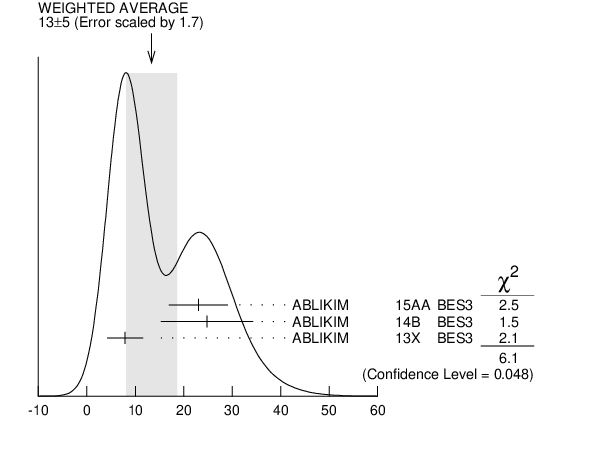#### ${{\boldsymbol X}{(4020)}^{\pm}}$ WIDTH

VALUE (MeV) EVTS DOCUMENT ID TECN CHG  COMMENT
 $\bf{ 13 \pm5}$ OUR AVERAGE  Error includes scale factor of 1.7. See the ideogram below.
$23.0$ $\pm6.0$ $\pm1.0$ 116 1
 2015 AA
BES3 0 ${{\mathit e}^{+}}$ ${{\mathit e}^{-}}$ $\rightarrow$ ( ${{\mathit D}^{*}}{{\overline{\mathit D}}^{*}}$ )${}^{0}{{\mathit \pi}^{0}}$
$24.8$ $\pm5.6$ $\pm7.7$ 401 1
 2014 B
BES3 $\pm{}$ ${{\mathit e}^{+}}$ ${{\mathit e}^{-}}$ $\rightarrow$ ( ${{\mathit D}^{*}}{{\overline{\mathit D}}^{*}}$ )${}^{+-}{{\mathit \pi}^{\mp}}$
$7.9$ $\pm2.7$ $\pm2.6$ 253 1
 2013 X
BES3 $\pm{}$ ${{\mathit e}^{+}}$ ${{\mathit e}^{-}}$ $\rightarrow$ ${{\mathit \pi}^{+}}{{\mathit \pi}^{-}}{{\mathit h}_{{c}}}$
 1 Neglecting interference between the ${{\mathit X}{(4020)}}$ and non-resonant continuum.${{\mathit X}{(4020)}^{\pm}}$ WIDTH (MeV)
References:
 ABLIKIM 2015AA
PRL 115 182002 Observation of a Neutral Charmoniumlike State ${{\mathit Z}_{{c}}{(4025)}^{0}}$ in ${{\mathit e}^{+}}$ ${{\mathit e}^{-}}$ $\rightarrow$ (${{\mathit D}^{*}}{{\overline{\mathit D}}^{*}}){}^{0}{{\mathit \pi}^{0}}$
 ABLIKIM 2014B
PRL 112 132001 Observation of a Charged Charmoniumlike Structure in ${{\mathit e}^{+}}$ ${{\mathit e}^{-}}$ $\rightarrow$ ( ${{\mathit D}^{*}}{{\overline{\mathit D}}^{*}}){}^{+-}{{\mathit \pi}^{\mp}}$ at $\sqrt {s }$ = 4.26 GeV
 ABLIKIM 2013X
PRL 111 242001 Observation of a Charged Charmoniumlike Structure ${{\mathit Z}_{{c}}{(4020)}}$ and Search for the ${{\mathit Z}_{{c}}{(3900)}}$ in ${{\mathit e}^{+}}$ ${{\mathit e}^{-}}$ $\rightarrow$ ${{\mathit \pi}^{+}}{{\mathit \pi}^{-}}{{\mathit h}_{{c}}}$